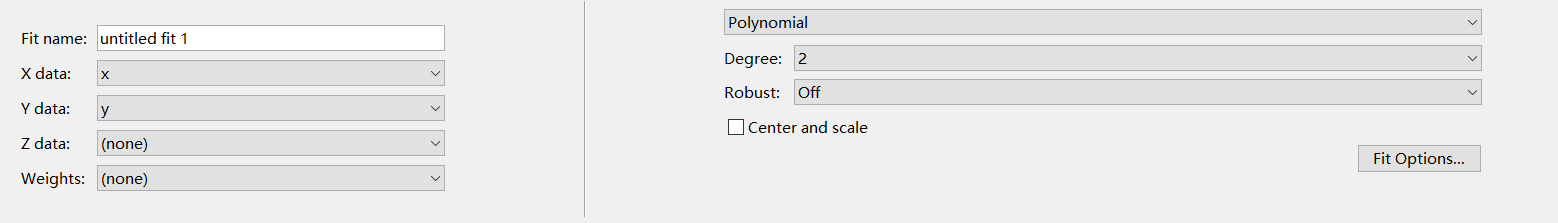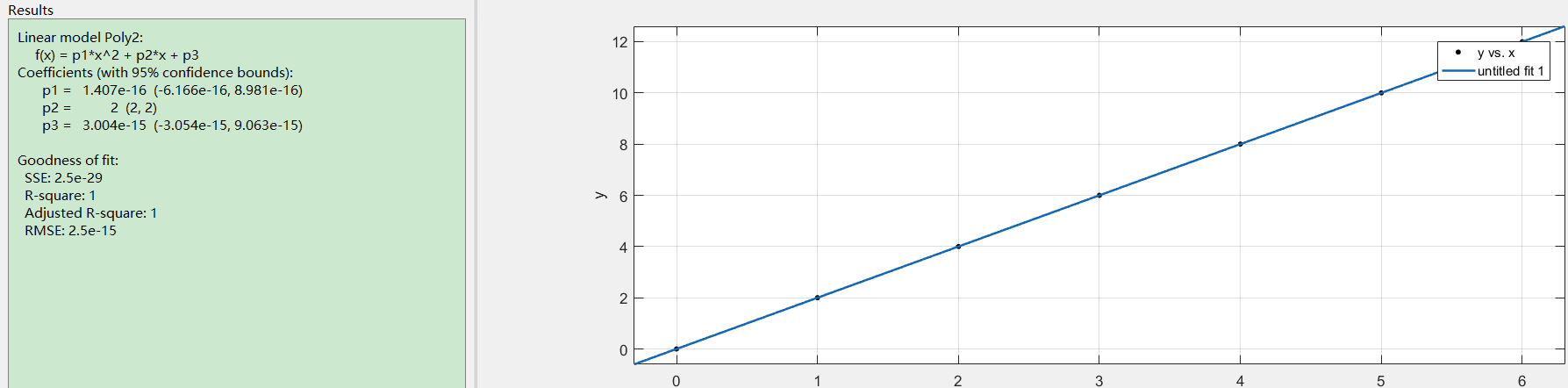• matlab拟合曲线 matlab拟合曲线 matlab拟合曲线
• ## MATLAB拟合曲线

千次阅读 2019-05-01 13:16:25
听别人说MATLAB拟合的时候特别方便，所以就测试了一下，果真方便，现在将大致的步骤罗列如下。 1.用一个矩阵表示一个自变量的取值； 2. 用另外一个矩阵表示因变量的取值； 3. 检查矩阵两个矩阵的数据元素个数...
    最近做一个实验 ，代码中需要找出数据之间的函数关系，所以需要把数据进行拟合，找到关系式。听别人说MATLAB做 拟合的时候特别方便，所以就测试了一下，果真方便，现在将大致的步骤罗列如下。

1.用一个矩阵表示一个自变量的取值；
2. 用另外一个矩阵表示因变量的取值；
3. 检查矩阵两个矩阵的数据元素个数是否相同（一般都是一个因变量对应一个自变量）
4. 调用语句 cftool
5. 在出现的窗口里面将 x data 与  y data 对应的矩阵进行链接
6. 选择要拟合的数学模型 ，如傅立叶，多项式
7. 等待一会儿，就出来了
举个例子：
x  取值 0  1 2 3 4 5 6
y  取值 0  2 4 6 8 10 12
在matlab 里面敲对应的语句为
x=[0 1 2 3 4 5 6];
y=[0 2 4 6 8 10 12];
cftool
在窗口里面选择如下：结果如下：展开全文• >> cftool >> y=[1.19 2.49 2.98 3.35 3.70 3.96]y = 1.1900 2.4900 2.9800 3.3500 3.7000 3.9600>> x=[0 0.2 0.4 0.6 0.8 1.0]x = 0 0.2000 0.4000 0.6000 0.80
>> cftool
>>  y=[1.19 2.49    2.98    3.35    3.70    3.96]

y =

1.1900    2.4900    2.9800    3.3500    3.7000    3.9600

>> x=[0 0.2 0.4 0.6 0.8 1.0]

x =

0    0.2000    0.4000    0.6000    0.8000    1.0000

>>  y=[1.19 2.49    2.98    3.35    3.70    3.96]

y =

1.1900    2.4900    2.9800    3.3500    3.7000    3.9600

>> 
展开全文• ## MATLAB拟合曲线

千次阅读 2016-08-03 21:28:49
今天想帮朋友做一个简单的数据拟合，然后画图，结果发现网上都没有现成的模板，只能自己摸索了，最后搜到了一个简单的方法，将多条曲线放到一个图上。 以下是数据：X1 = 0 0.8800 4.0000 1.0400 8.0000 1.0200 ...
今天想帮朋友做一个简单的数据拟合，然后画图，结果发现网上都没有现成的模板，只能自己摸索了，最后搜到了一个简单的方法，将多条曲线放到一个图上。
以下是数据：

X1 =
0    0.8800
4.0000    1.0400
8.0000    1.0200
12.0000    1.1000
x1 =
0     4     8    12
y1 =
0.8800    1.0400    1.0200    1.1000
X2 =
0    2.1600
4.0000    3.0500
8.0000    3.2400
12.0000    3.9500
x2 =
0     4     8    12
y2 =
2.1600    3.0500    3.2400    3.9500
X3 =
0    4.2900
4.0000    3.2300
8.0000    2.8600
12.0000    3.3200
16.0000    4.7900
x3 =
0     4     8    12    16
y3 =
4.2900    3.2300    2.8600    3.3200    4.7900

注意画图的x与y都必须是行向量
首先做拟合，这个很简单，命令行中输入cftool就可以了，曲线类型选择smoothingspline平滑曲线，选择x1，y1，就可以得到第一条拟合曲线了，但是怎么样将多条拟合曲线画在一张图上呢，首先在cftool中选择fit->new fit，然后依次得到第二条与第三条拟合曲线，然后在file->generate code中得到画图的代码，如下：

function [fitresult, gof] = createFits1(x1, y1, x2, y2, x3, y3)
%CREATEFITS1(X1,Y1,X2,Y2,X3,Y3)
%  Create fits.
%
%  Data for 'untitled fit 1' fit:
%      X Input : x1
%      Y Output: y1
%  Data for 'untitled fit 3' fit:
%      X Input : x2
%      Y Output: y2
%  Data for 'untitled fit 4' fit:
%      X Input : x3
%      Y Output: y3
%  Output:
%      fitresult : a cell-array of fit objects representing the fits.
%      gof : structure array with goodness-of fit info.
%

%  Auto-generated by MATLAB on 12-Oct-2016 20:52:56

%% Initialization.

% Initialize arrays to store fits and goodness-of-fit.
fitresult = cell( 3, 1 );
gof = struct( 'sse', cell( 3, 1 ), ...
'rsquare', [], 'dfe', [], 'adjrsquare', [], 'rmse', [] );

%% Fit: 'untitled fit 1'.
[xData, yData] = prepareCurveData( x1, y1 );

% Set up fittype and options.
ft = fittype( 'smoothingspline' );

% Fit model to data.
[fitresult{1}, gof(1)] = fit( xData, yData, ft );

% Plot fit with data.
figure( 'Name', 'untitled fit 1' );
h = plot( fitresult{1}, xData, yData );
legend( h, 'y1 vs. x1', 'untitled fit 1', 'Location', 'NorthEast' );
% Label axes
xlabel x1
ylabel y1
grid on

%% Fit: 'untitled fit 3'.
[xData, yData] = prepareCurveData( x2, y2 );

% Set up fittype and options.
ft = fittype( 'smoothingspline' );

% Fit model to data.
[fitresult{2}, gof(2)] = fit( xData, yData, ft );

% Plot fit with data.
figure( 'Name', 'untitled fit 3' );
h = plot( fitresult{2}, xData, yData );
legend( h, 'y2 vs. x2', 'untitled fit 3', 'Location', 'NorthEast' );
% Label axes
xlabel x2
ylabel y2
grid on

%% Fit: 'untitled fit 4'.
[xData, yData] = prepareCurveData( x3, y3 );

% Set up fittype and options.
ft = fittype( 'smoothingspline' );

% Fit model to data.
[fitresult{3}, gof(3)] = fit( xData, yData, ft );

% Plot fit with data.
figure( 'Name', 'untitled fit 4' );
h = plot( fitresult{3}, xData, yData );
legend( h, 'y3 vs. x3', 'untitled fit 4', 'Location', 'NorthEast' );
% Label axes
xlabel x3
ylabel y3
grid on


根据这一段代码其实就可以发现我们其实根本不需要打开cftool，直接写代码就可以得到拟合曲线，通过hold on就可以很轻易的将不同的曲线画在同一张图上了，具体代码如下：

ft = fittype( 'smoothingspline' );
[fit1, gof] = fit( x1', y1', ft );
[fit2, gof] = fit( x2', y2', ft );
[fit3, gof] = fit( x3', y3', ft );
h=plot( fit1, x1, y1 );
set(h,'Color','g')
hold on
h1=plot( fit2, x2, y2 );
set(h1,'Color','r')
hold on
h2=plot( fit3, x3, y3 );
set(h2,'Color','b')


通过set更改曲线的颜色，注意Color要大写，小写只会改数据点的颜色
其他的设置像图例坐标轴标题什么的百度一下就好
展开全文数据
• 本资源是有关数学建模中曲线拟合的问题，包括ppt文档和具体的详细源代码。
• Matlab拟合曲线，并显示曲线上最大的点对应的x,y值 x=[292 298 303 307 311 316 325 329 334 339 344]; y=[3706 4778 5378 6560 7464 8111 8065 7496 5994 4706 3317]; plot(x,y,'o') p=polyfit(x,y,6)%拟合...
Matlab拟合曲线，并显示曲线上最大的点对应的x,y值
x=[292 298 303 307 311 316 325 329 334 339 344];
y=[3706 4778 5378 6560 7464 8111 8065 7496 5994 4706 3317];
plot(x,y,'o')
p=polyfit(x,y,6)%拟合曲线 6次项
x1=292:1:344;
y1=polyval(p,x1);
plot(x,y,'o',x1,y1,'-b')
maxY=max(y1)
indexX=find(y1==maxY);%查找最大值所在的索引
x_value=x1(29)

展开全文plot x
• 需要用到matlab拟合曲线中的smoothing spline功能，同时origin画的图又比较好看且可以后续做多条切线，因此记录下matlab与origin联合使用的方法，并加上用origin的tangent插件做曲线多条切线的方法。
• 1.创建两个列表； 2.输入cftool调出下图窗口，并修改相应的值； 3.点击 print to figure 导出图片；
• 实验目的  学习由实际问题去建立数学模型的全过程;  训练综合应用数学模型、...少年易学老难成,一寸...+86-551-5690963 5690964 使用 MATLAB 曲线拟合工具箱做曲线拟合胡庆婉(曲靖师范学院 数学与信息科学学...
• 什么是曲线拟合所谓的曲线拟合，就是使用某一个模型（或者称为方程式），将一系列的数据拟成平滑的曲线，以便观察两组数据之间的内在联系，了解数据之间的变化趋势。曲线拟合的作用在数据分析时，我们有时需要通过已...
• 输入数据 做数据曲线拟合，当然该有数据，本经验从以如下数据作为案例。 ...这一步就是将你要拟合的数据添加到curve fitting程序中，同时给你拟合...常见的拟合曲线有多项式的、指数的、对数的等等。curve fitt...
• 如何用matlab拟合幂律分布，怎样将拟合值和实际值进行对比，放在一个图中，又如何检验实际数据是否符合拟合函数。如果不符合，如何来直接判断实际数据服从什么样的函数分布呢 在MATLAB里，对数据进行...幂律分布
• 收集的问题： 如何用matlab来拟合幂律分布，怎样将拟合值和实际值进行对比，放在一个图中，...如果是不需要得到拟合曲线的函数，只是把这些点利用一些光滑曲线连接，建议使用三次样条函数spline（）进行插值即可。
• 一、（1）已知数据如下：x 1.0 1.4 1.8 2.2 2.6 y 0.931 0.473 0.297 0.224 0.168 求形如拟合函数。解：y1=polyfit(x,y,N)函数的说明：这里函数polyfit第一个参数传递的是拟合数据的自变量，第二个参数是因变量，第...
• 好了，现在我们可以把数据和拟合曲线画在同一张图上了： gnuplot> set xrange [8:16] gnuplot> set yrange [-5:105] gnuplot> unset key gnuplot> set xlabel "Laser Pulse Energy (μJ)" gnuplot> set ylabel ...
• CODE:Variable x1,x2,y;Function y=a1*a2*x1*exp(x2-3.64)/(1+ (1+a2*x1)*exp(x2-3.64) );Data;9.7134 8.0000206.59174.0793 8.0000181.03781.6373 8.0000130.75210.4996 8.0000 97.27970.0617...
• 该楼层疑似违规已被系统折叠隐藏此楼查看此楼程序...五阶拟合函数是f5=0.0049664*T^5-49.4903*T^4+197266.7483*T^3-393146782.4823*T^2+391762509341.9921*T-156152262895446.5;dc1=diff(0.0049664*T^5-49.4903*T^4+...
• 该楼层疑似违规已被系统折叠隐藏此楼查看此楼程序...五阶拟合函数是f5=0.0049664*T^5-49.4903*T^4+197266.7483*T^3-393146782.4823*T^2+391762509341.9921*T-156152262895446.5;dc1=diff(0.0049664*T^5-49.4903*T^4+...
• 网上教程有好有坏，我自己总结了一个笔记 目录 ...9.如何使用拟合曲线 1.打开方式 输入cftool 或者 2. 打开界面 3.输入数据 4.选择拟合方式 Custom Equations：用户自定义的函数类型...
• matlab曲线拟合-MATLAB曲线拟合向导.doc 自己下载的一些曲线插值，拟合的资料，希望对大家有所帮助
• 利用MATLAB曲线拟合工具箱求离散点的拟合曲线，陈巍，，曲线拟合是数值分析中的一种普遍且重要的方法，求解拟合曲线的方法也有很多.本文主要介绍利用MATLAB曲线拟合工具箱对离散数据点做�...

# matlab拟合曲线matlab 订阅